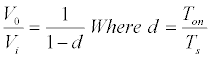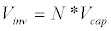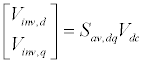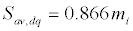Reach Us+44-1522-440391
On-Line Maximum Power Point Tracking for Photovoltaic System Grid Connected Through DC-DC Boost Converter and Three Phase Inverter | OMICS International
Journal of Fundamentals of Renewable Energy and Applications
All submissions of the EM system will be redirected to Online Manuscript Submission System. Authors are requested to submit articles directly to Online Manuscript Submission System of respective journal.

# On-Line Maximum Power Point Tracking for Photovoltaic System Grid Connected Through DC-DC Boost Converter and Three Phase Inverter

MMA Mahfouz*

Electrical Power and Machines Department, Faculty of Engineering, Helwan University, Cairo, Egypt

Corresponding Author:
Mahfouz MMA
Faculty of Engineering
Electrical Power and Machines Department
Helwan University, Cairo, Egypt
Tel: +20225197368
E-mail: [email protected]

Received date: June 01, 2015; Accepted date: June 12, 2015; Published date: June 19, 2015

Citation: Mahfouz MMA (2015) On-Line Maximum Power Point Tracking for Photovoltaic System Grid Connected Through DC-DC Boost Converter and Three Phase Inverter. J Fundam Renewable Energy Appl 5:165. doi:10.4172/2090-4541.1000165

Copyright: © 2015 Mahfouz MMA. This is an open-access article distributed under the terms of the Creative Commons Attribution License, which permits unrestricted use, distribution, and reproduction in any medium, provided the original author and source are credited.

Visit for more related articles at Journal of Fundamentals of Renewable Energy and Applications

#### Abstract

Photovoltaic solar electricity and solar thermal has the highest potential of all the renewable energies, since solar energy is a practically unlimited resource and available everywhere. These days photovoltaic energy has the potential to play an important role in the transition towards a sustainable energy supply system, to cover a significant share of the electricity needs, and is expected to be one of the key energy technologies of this century. This paper presents a grid-connected photovoltaic (PV) system with the functionality of on-line Maximum Power Point Tracking (MPPT). The integration topology is based on two-stage power conditioning modules, boost converter and three phase voltage source inverter. Therefore, the proposed MPPT algorithm is implemented to the boost converter to enable PV arrays to operate at maximum power point during variable climate and irradiation conditions. Fuzzy logic algorithm (MPPT) technique control is applied for on-line adapting the boost converter duty cycle. The Boost output is feeding the two level PWM inverter DC link. Adoringly, the Inverter modulation depth is tuned to have constant AC voltage and frequency for grid integration requirements. In addition, the three-phase output in phase with the voltage to optimize the PV power utilization .The results show that proposed on-line fuzzy algorithm performance has a fast response, stable and reliable to have MPP for the PV system during the different irradiation.

#### Keywords

Renewable energy; Photovoltaic; Maximum power point tracking (MPPT); Fuzzy logic; DC-DC converter; Three phase PWM inverter

#### Introduction

The growing energy demand coupled with the possibility of reduced supply of conventional fuels, along with growing concerns about environmental preservation, has driven research and development of alternative energy sources that are cleaner, renewable and produce little environmental impact . Among the alternative sources, the electrical energy from PV is currently regarded as the natural energy source distributed over the earth and participates as a primary factor of all other processes of energy production on earth. Moreover, although the phenomena of reflection and absorption of sunlight by the atmosphere, it is estimated that solar energy incident on the surface of earth is of the order of ten thousand times greater than the world energy consumption . In this context, the concept of distributed energy generation, became a real and present technical possibility, promotion various researches and standardizations in the world. Despite all the advantages presented by the generation of energy through the use of PV’s, the efficiency of energy conversion is currently low and the initial cost for its implementation is still considered high, and thus it becomes necessary to use techniques to extract the maximum power from these panels, to achieve maximum efficiency in operation. It should be noted that there is only one point of maximum power (MPP), and this varies according to climatic conditions. The photovoltaic power characteristics is nonlinear, which vary with the level of solar irradiation and temperature, which make the extraction of maximum power a complex task, considering load variations. To overcome this problem, several methods for extracting the maximum power have been proposed in literature [3,4], and a careful comparison of these methods can result in important information for the design of these systems. There are a lot of techniques are used for MPPT such as Fixed Duty Cycle, Constant Voltage, Perturb and Observe (P&O) and Modified P&O, Incremental Conductance (IC) and Modified IC, Ripple Correlation and System Oscillation methods, which are briefly described in this section [5,6].

This paper, target is to have an on-line efficient optimization (MPPT) algorithm using fuzzy logic technique. In the studied power system PV, integration to the grid is done by using two stages; the PV array output is connected to DC-DC converter followed by two level voltage sources VSPWM inverter to the grid. Maximum power point tracking (MPPT) techniques is used to control the DC converter to extract maximum output power from under a given weather condition. The DC converter is on-line continuously controlled to have the maximum power point operation for the PV array in despite of the possible changes either on the climate condition and/or in the load impedance. To achieve this paper’s target; the paper organized in the following sequences:

• Modeling of the PV–grid connected system for applying MPPT techniques using MATLAB/Simulink.

• Simulation of the PV–gird system during weather variation represented by an actual daily solar irradiation curve.

• The dynamic response of the algorithm is characterized by driving the PV system into its maximum power operation while ensuring that the injected current remains in phase with the grid voltage to achieve a unity power factor.

• Investigate the interactions between the PV system outputs and the grid.

Modeling of the PV-Grid Connected System

Power system under study single line diagram is illustrated in Figure 1. It consists of 22 kV interconnected network. The studied PV array is poly crystalline silicon each cell power maximum of 56 W and consists of 36 series cells per module with an open circuit 20.8 V, 16 series connected modules and 123 parallel with total maximum power rating of 100 kW is connected to the grid and to each other’s. The PV arrays are connected to the grid through DC/DC boost converter and DC/AC three-phase two-level inverter. After inversion, the arrays are interfaced to the grid through a transformer 220 V/22 kV of rated 125 kVA. A local load of active power 50 kW is connected to the grid integration bus at PV point of common coupling.

PV array

The equivalent circuit model of a PV cell is needed to simulate its real behavior using the physics of p-n junctions; a cell can be modeled as a DC current source in parallel with diode that represents the most effective current escaping due to diffusion and charge recombination mechanisms. Two resistances, series and shunt are included to m represent the contact resistances and the internal PV cell resistance respectively. These two resistance values are usually obtained from measurements or applying the curve fitting technique based on the cell I-V characteristic . The equivalent circuit model of a PV cell is needed to simulate its real behavior using the physics of p-n junctions, a cell can be modeled as a DC current source in parallel with diode that represents the most effective current escaping due to diffusion and charge recombination mechanisms. Two resistances, series and parallel, are included to represent the contact resistances and the internal PV cell resistance respectively. These two resistance values are usually obtained from measurements or applying the curve fitting technique based on the cell I-V characteristic . PV cells are grouped in larger units called PV modules, which are further interconnected in a parallelseries configuration to form PV arrays or PV generators and the voltage and power of the PV module are depending on the number module No. of series and parallel cells. A grid-connected PV solar system is to transfer the maximum power obtained from the PV panel into the grid independently of the climatic conditions. Therefore, the use of an appropriate electronic interface with maximum power point tracking (MPPT) capabilities is required. The output power of the PV cell has a nonlinear function with irradiance and temperature. Therefore, the power of the PV array changes continuously and consequently the PV operating point must change to maximize the energy produced by adjusting array voltage to its calculated maximum value at certain irradiation and temperature. Figure 2 shows the used power- voltage characteristics of the used PV array at different climate conditions and the target maximum power points for the proposed control system.

Figure 2: PV model characteristics; a) Different radiation and b) Different temperature.

Boost chopper

A buck boost converter is a power converter with an output DC voltage greater or less than its input DC voltage depending on its duty cycle (d) as illustrated in Figure 3. By implementing the PWM technique on the buck boost converter, a stable output voltage from a non-stable input voltage can be obtained by changing the duty cycle (d) of the switched input pulse. The buck boost converter with a switching period (Ts), and on-period of (Ton) the relationship between output Vo and Vin voltage is governed by [9,10];(1)

After determining the value for VMPP, the DC/DC buck boost converter is switched to quickly force the array terminal voltage to its MPP. The fast switching action of the boost converter decouples the dynamics of the PV array due its changes in voltage or current from that of the DC link capacitor, which offers good performance under changing weather conditions. The other advantage of using the boost converter is to increase the array voltage to higher levels suitable for grid interconnection.

Three -phase voltage source PWM inverter

Among various modulation techniques, PWM is an attractive candidate due to efficient DC link voltage utilization, reducing commutation losses and total harmonic distortion THD. The proposed inverter is a three-phase, two-level DC/AC inverter using IGBTs due to their lower switching losses. Depending on the gating signals, the output terminal voltage is either equal to capacitor voltage or zero, and can be expressed by [11,12]:(2)

where: Vinv, N, and Vcap are terminal voltage, gating signal and capacitor voltage, respectively. N takes the value of either one or zero. In the two-level inverter the switching of the upper and lower IGBT generates the output voltages with positive and negative levels (+Vdc and -Vdc). The inverter is generally connected to the network at the PCC through the equivalent inductance and resistance, which represent the impedance of the coupling transformer. The magnetizing inductance of the step-up transformer can also be taken into consideration through a mutual equivalent inductance. The aim of the control strategy is to regulate the current output from the inverter to follow a specified reference value. This can be achieved by transforming the three phase output currents of the inverter to the rotating reference frame (dq0). The relation between the DC side voltage Vdc and the generated AC voltage Vinv can be described through the matrix in the dq-frame Sav, dq, as given by:(3)

where(4)

where; mi is the inverter modulation index; and Vdc is equal to the Boost converter output (Vo).

#### Proposed Control Topology

This section describe two main parts the proposed global PV MPPT control scheme philosophy and secondly the proposed fuzzy logic algorithm to apply the MPPT concept. As shown in Figure 4, the block diagram of the global MPPT concept. A photovoltaic array is used to convert sunlight into DC current. The output of the array is connected to a boost DC converter that is used to perform MPPT functions and increase the array terminal voltage to a higher value so it can be interfaced to the grid at 22 kV. The DC converter controller is used to perform these two functions. A DC link capacitor is used after the DC converter and acts as a temporary power storage device to provide the voltage source inverter with a steady flow of power. The capacitor’s voltage is regulated using a DC link controller that balances input and output powers of the capacitor. The voltage source inverter is controlled in the rotating dq frame is to inject a controllable three phase AC current into the grid. To achieve unity power factor operation, current is injected in phase with the grid voltage. A phase locked loop (PLL) is used to lock on the grid frequency and provide a stable reference synchronization signal for the inverter control system, which works to minimize the error between the actual injected current and the reference current obtained from the DC link controller. A load is connected to the grid to simulate some of the loads that are connected to a distribution system network.

Proposed MPPT fuzzy algorithm

There are for Fuzzy logic MPPT algoritm has three sequential stages; fuzzification, rule based on the look-up table and finally the defuzzification. During fuzzification process, control variable are been converted from a numerical value to a linguistic representation . In this paper, Mamadani fuzzy type is used as shown in Figure 5a with two inputs. These inputs are the error between the actual PV power and the maximum reference power cross pounding to the synchronized irradiation and the change of this error. The fuzzy controller output is the DC boost duty cycle. The fuzzy surface to show the ranges and the relation between the inputs and the output memberships is illustrated in Figure 5b. Twenty-one rules between the inputs to achieve the requested output target. This target is to minimize the error and the rate of error to zero to achieve the tracking for PV power equal to the MPP.

#### Simulation Results

Studied power system for PV grid connected system at a daily irradiation and temperature of 25˚C using MATLAB/Simulink program is simulated in this part to verify the dynamic and steady state response of the proposed fuzzy MPPT control system. In besides that monitoring of the DC and AC voltages and currents in the PV array and the associated power conditioning system. Figure 6a present the irradiation data which is typically get from the Arab Organization for Industrialization (AOI) Cairo irradiation records on summer 2011 at temperature of 25˚C to verify the performance at Cairo irradiation conditions. Figure 6b shows that the PV output after applying the proposed control to have MPPT is so closely and equal to the target calculated maximum power from the (Power /voltage) curve of the PV array at the different solar irradiation which already shown in Figure 2a. While Figure 6c illustrates the PV array dc voltage changes during the different conditions of radiation. The DC-DC Boost converter performance illustrate in Figure 7. The duty cycle behavior is shown in Figure 7a, it is changes to increase the Boost output voltage during the decreasing of the irradiation and its values ranged from 0.45 to 0.6. While Figure 7b represents the output Vdc with its reference values, it shows that the Vdc is follows the Vdc reference values that validate the proposed control technique. The second stage of voltage conditioning unit, three-phase voltage source inverter using pulse width modulation parameters are shown in Figure 8. In Figure 8a the modulation depth performances is illustrated while the output three phase voltage and current are shown in Figure 8b. It shows that the modulation depth is dynamically interacted with fast response to have a constant AC voltage output, which is essential for PV grid synchronizing. Also the three phase injected current is in phase with output voltage to have maximum utilization from the PV system. The three power components, load power, PV output and grid power sharing at the point of common coupling at the grid Bus in presented at Figure 9. As shown, the load power is constant while the way of supplying this load is changed depending on the PV system output. When the irradiation is high; the PV power is greater than the load and the extra power is supplied to the grid. While if the irradiation decreased, and the PV output power is less than the load, in this case the load is complimentary supplied from both and the PV.

Figure 6: irradiation and PV array output; a) irradiation, b) PV power and C) PV voltage.

#### Conclusion

Modeling of grid connected photovoltaic system elements with two sequential stages of power electronic modules; DC-DC boost converter and three-phase two level voltage source PWM inverter was presented. Design of on-line control system to have Maximum power point tracking from the PV array using Fuzzy Logic Controller algorithms was introduced. The results show that the proposed online fuzzy logic controller of the MPP offers clearly short settling time, accurate tracking the reference voltage during all the weather conditions. In addition, the proposed MPPT control algorithm performance is stable, fast, reliable and adaptive to be implemented for PV micro grids and high voltage grid integration.

#### References

Select your language of interest to view the total content in your interested language

### Article Usage

• Total views: 14400
• [From(publication date):
July-2015 - Aug 25, 2019]
• Breakdown by view type
• HTML page views : 10382
• PDF downloads : 4018

## Post your commentCan't read the image? click here to refresh
###### Peer Reviewed Journals

Make the best use of Scientific Research and information from our 700 + peer reviewed, Open Access Journals

International Conferences 2019-20

Meet Inspiring Speakers and Experts at our 3000+ Global Annual Meetings

Top